﻿ Comsol Tutorials DipolHome > Tutorials > Comsol Tutorials > Comsol Tutorials Dipol

Programme

File Backupper

Digitize Plot To Data

Scrabbler

Tutorials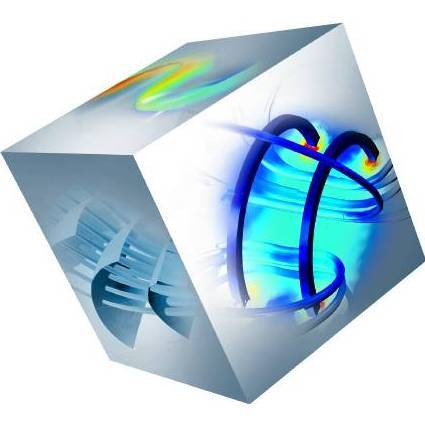Comsol 4.2 Tutorials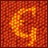Gwyddion Tutorials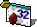VB 6.0 TutorialsTutorials: sonstComsol Tutorials Dipol

﻿COMSOL 4.2
- EasyTutorials

COMSOL is a finite element simulation software, witch can basically simulate anything you want: it simulates heat transfer through complex structures, photonic crystals on the nanoscale, mechanical bending of beams, flow of fluids, electrochemical processes, plasma physics and many more.

These tutorials were made, because the author found a lack of simple COMSOL examples on the web or on youtube. The tutorials from the COMSOL webpage are way to elaborate and to complicated. Very important and often simple things such as extracting data from the simulation are neglected. In the COMSOL tutorials it is shown for example how the microwave heating of a potato can be simulated, but it is missed out how you can use this simulation to extract a heat profile and use the data in an other software to make another calculation or a simple plot.

The Tutorials on this webpage were created to get you going with COMSOL 4.2. The aim is to show basic principles, how to use the tools, how to extract data and so on. For each field of simulation you should however, have a good knowledge about the theory.

COMSOL 4.2 - EasyTutorials 01 - Simple Dipole

This is a tutorial for COMSOL 4.2. It demonstrates a simple example of how to create and simulate an electric dipole in air.
Used field and intensity plots:

sqrt(emw.Poavx^2+emw.Poavy^2+emw.Poavz^2)
sqrt(Ex^2+Ey^2)

Obtained result picture: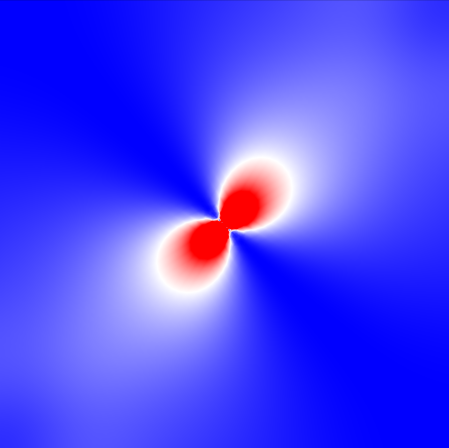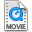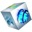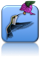Home      Impressum